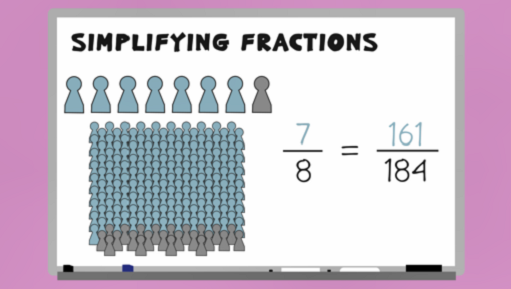Everyday maths 2 (Wales)

Start this free course now. Just create an account and sign in. Enrol and complete the course for a free statement of participation or digital badge if available.

Free course

# 7.1 Simplifying fractions

Watch the video below which looks at how to simplify fractions before having a go yourself in Activity 14.Skip transcript

#### Transcript

In this video, you'll look at how to simplify fractions. You'll be used to seeing the results of company surveys given as fractions: '7/8 people say they were satisfied with our customer service'. This is an example of a fraction in its simplest form. It could be that 184 people were surveyed and that 161 of them responded to say they were satisfied. Although they are equivalent fractions, you can see that the fraction '7/8 people' is a lot more user friendly than saying 161/184 people responded to say they were satisfied.

If you're asked to give an answer as a fraction in its simplest form, you firstly find a number that you can divide both parts of the fraction by, and divide it. For example, to simplify the fraction 12/18, you can divide both the top and the bottom number by 2, to give 6/9.

Keep going until you can't find a number that you can divide both parts of the fraction by. The fraction 6/9 can be divided again, this time by 3, to get 2/3. This is now the fraction in its simplest form.

Now let's simplify the fraction 40/120. Remember, find a number that you can divide both parts of the fraction by, and keep going until there is no longer a number that works. So, divide the top and bottom by 10. Divide the top and bottom by 2. Divide the top and bottom by 2. This is the fraction in its simplest form.

End transcript

Interactive feature not available in single page view (see it in standard view).

## Activity 14: Simplifying fractions

Show the following fractions in simplest form, where possible:

• a.

• b.

• c.

• d.

• e.

• f.

• a. =

• b. =

• c. =

• d. =

• e. can’t be simplified

• f. =

Next you’ll look at expressing a quantity of an amount as a fraction.

FSM_2_CYMRU#### Find out more

*Eligibility rules apply for financial support.